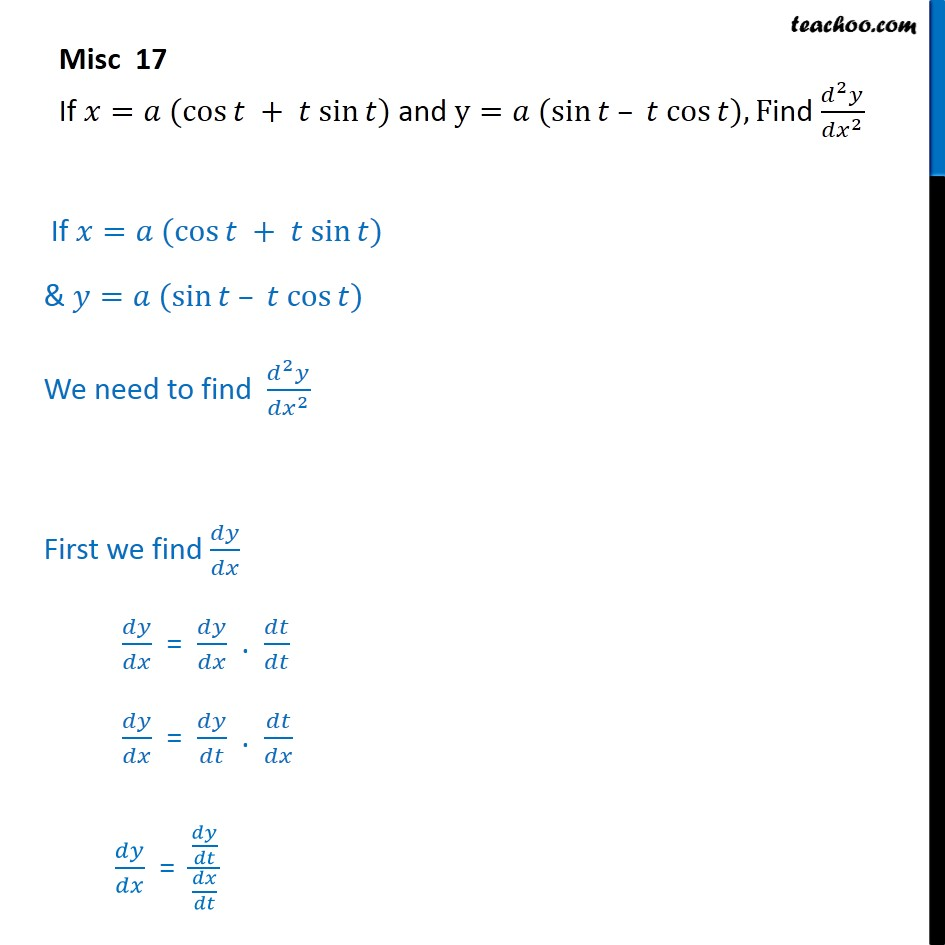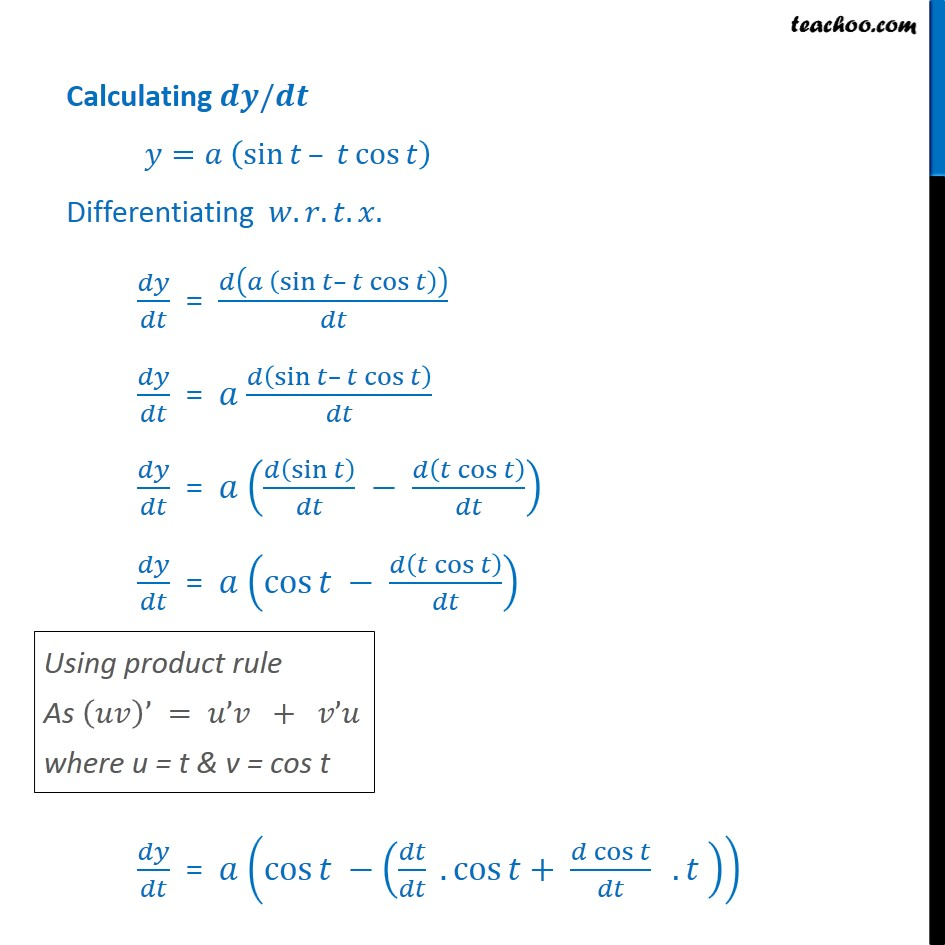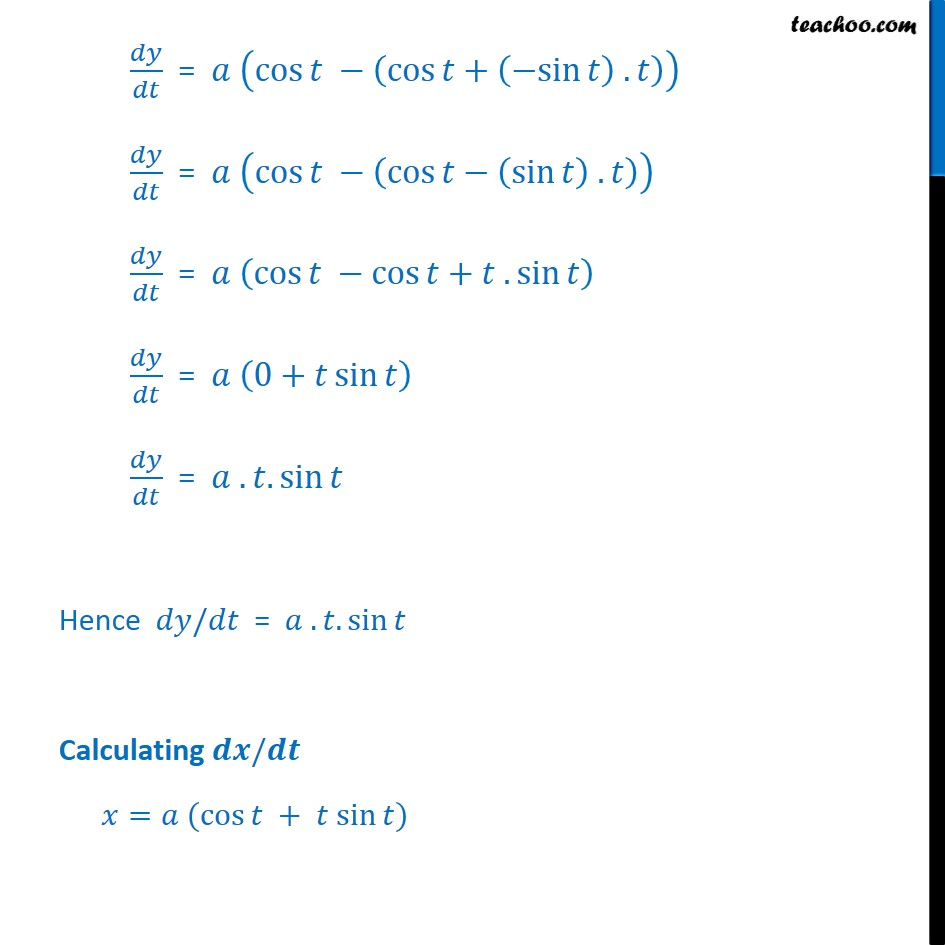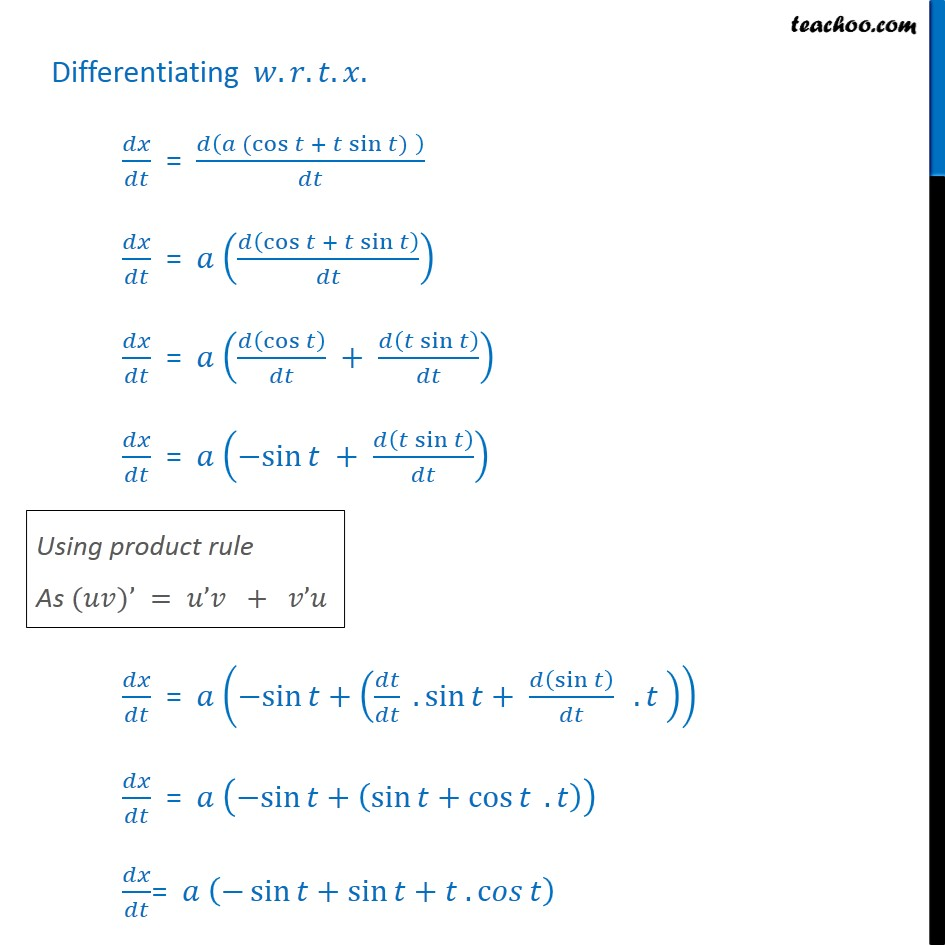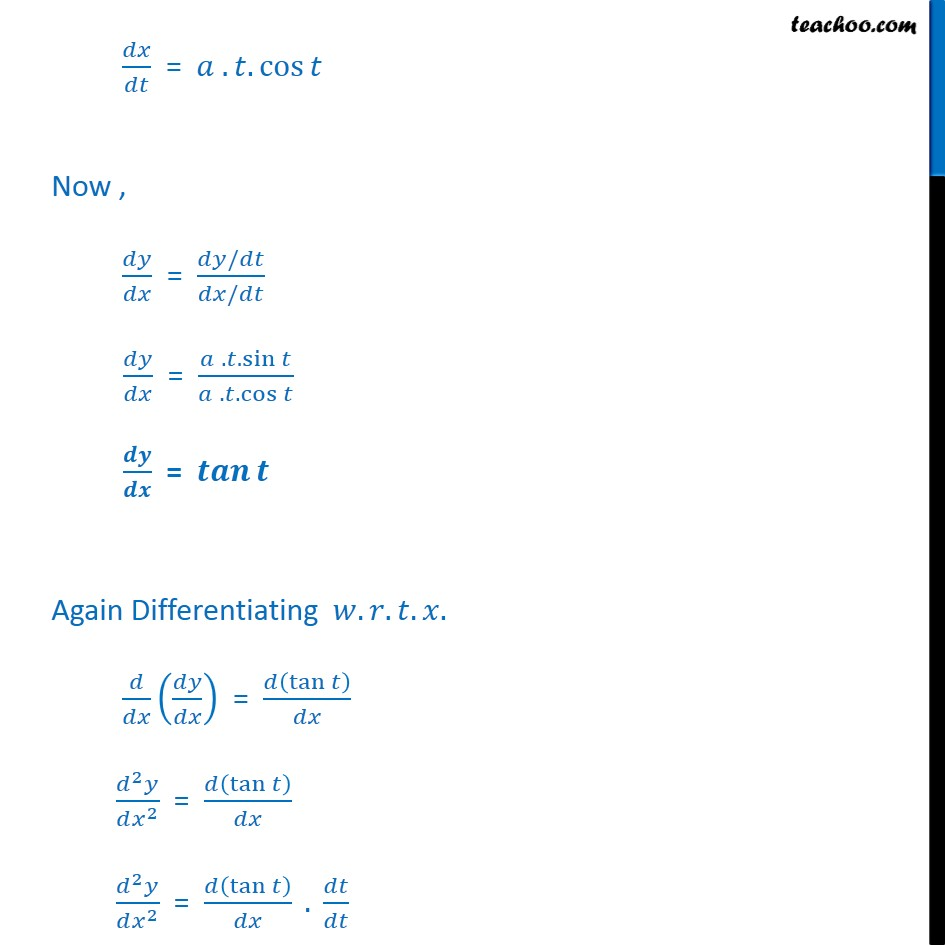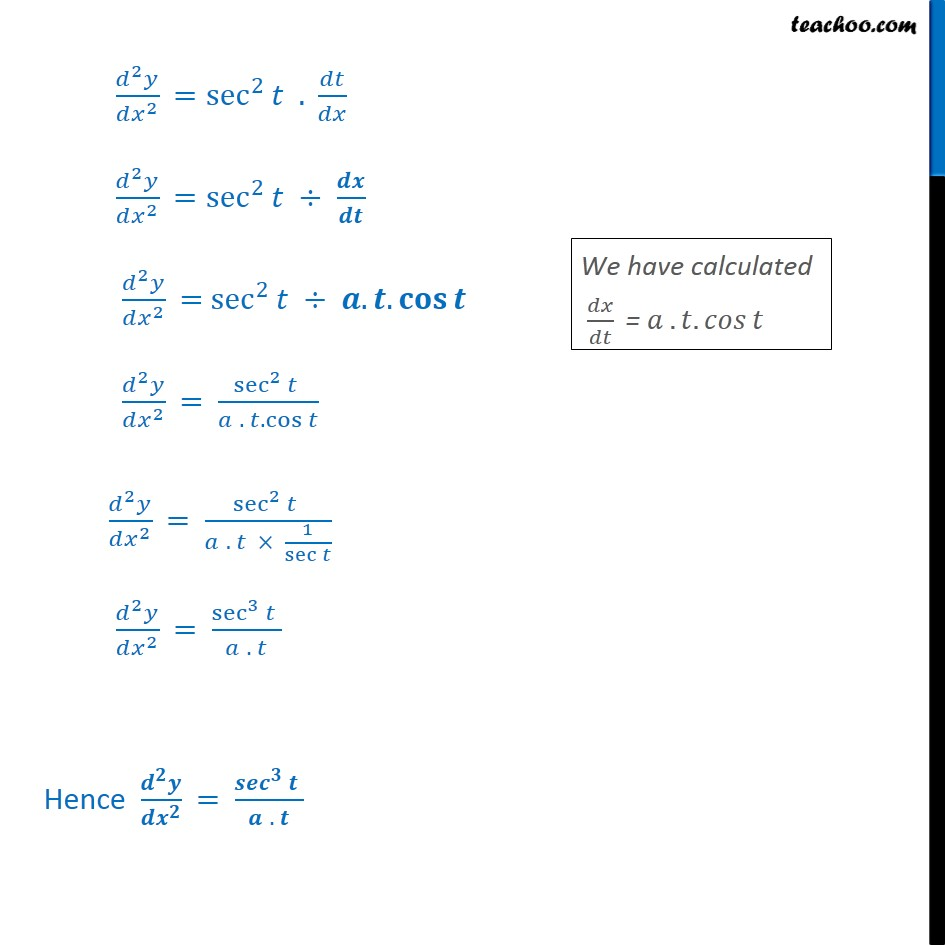1. Chapter 5 Class 12 Continuity and Differentiability
2. Serial order wise
3. Miscellaneous

Transcript

Misc 17 If = (cos + sin ) and y= (sin cos ), Find 2 2 If = (cos + sin ) & = (sin cos ) We need to find 2 2 First we find = . = . = Calculating / = sin cos Differentiating . . . . = sin cos = sin cos = sin cos = cos cos = cos . cos + cos . = cos cos + sin . = cos cos sin . = cos cos + . sin = 0+ sin = . . sin Hence / = . . sin Calculating / = (cos + sin ) Differentiating . . . . = (cos + sin ) = cos + sin = cos + sin = sin + sin = sin + . sin + sin . = sin + sin + cos . = sin + sin + . c = . . cos Now , = / / = . . sin . . cos = Again Differentiating . . . . = tan 2 2 = tan 2 2 = tan . 2 2 = sec 2 . 2 2 = sec 2 2 2 = sec 2 . . 2 2 = sec 2 . . cos 2 2 = sec 2 . 1 sec 2 2 = sec 3 . Hence = .

Miscellaneous